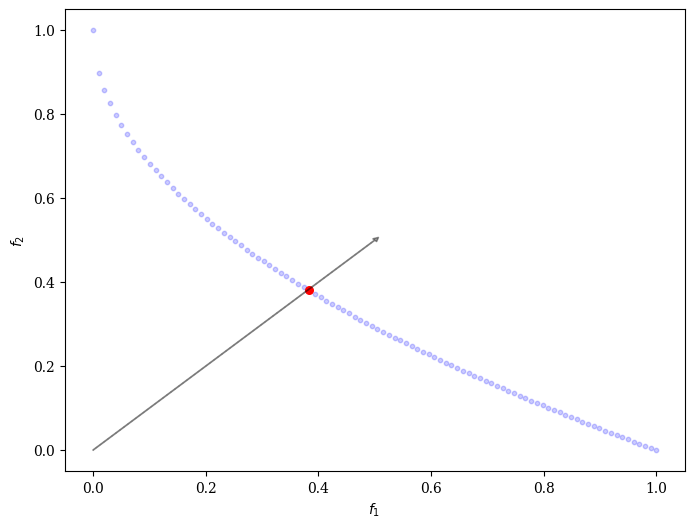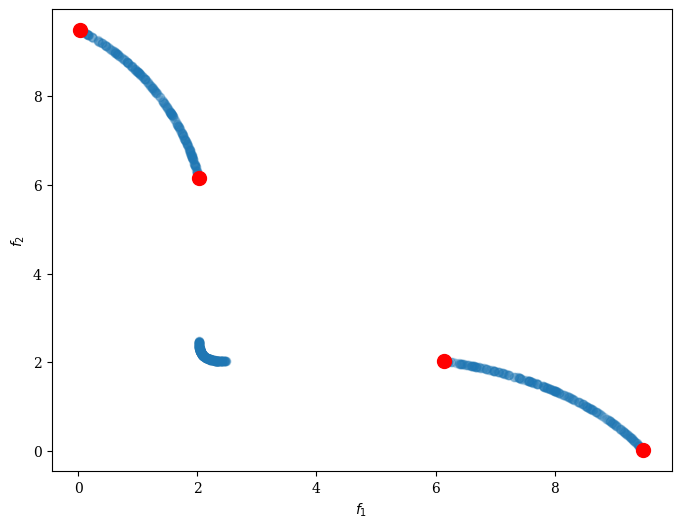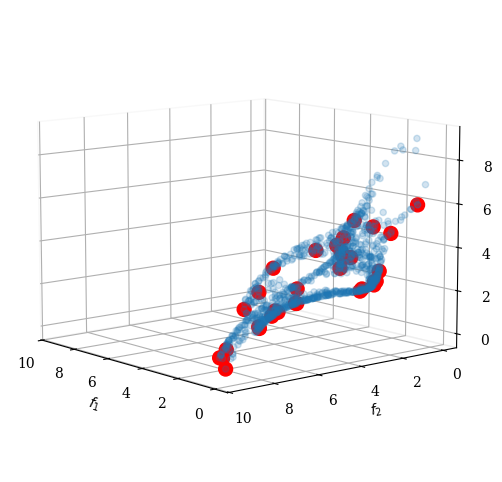Version: 0.5.0

# Multi-Criteria Decision Making (MCDM)¶

The focus of pymoo is on optimization methods itself. However, some basic multi-criteria decision making methods are available:

## Compromise Programming¶

We can use any scalarization method and use it for post-processing. Let us assume our algorithm has converged to the Pareto-front:

:

from pymoo.factory import get_problem

F = get_problem("zdt1").pareto_front()


Then, we initialize weights and our decomposition function:

:

from pymoo.factory import get_problem, get_visualization, get_decomposition
import numpy as np

weights = np.array([0.5, 0.5])
decomp = get_decomposition("asf")


We apply the decomposition and retrieve the best value (here minimum):

:

I = decomp.do(F, weights).argmin()
print("Best regarding decomposition: Point %s - %s" % (I, F[I]))

Best regarding decomposition: Point 38 - [0.38383838 0.38045308]


Visualize it:

:

from pymoo.factory import get_visualization

plot = get_visualization("scatter")
plot.do()
plot.apply(lambda ax: ax.arrow(0, 0, 0.5, 0.5, color='black',
plot.show()

:

<pymoo.visualization.scatter.Scatter at 0x7f4061d08f40>## Pseudo-Weights¶

A simple way to chose a solution out of a solution set in the context of multi-objective optimization is the pseudo-weight vector approach proposed in . Respectively, the pseudo weight $$w_i$$ for the i-ith objective function can be calculated by:

\begin{equation} w_i = \frac{(f_i^{max} - f_i {(x)}) \, /\, (f_i^{max} - f_i^{min})}{\sum_{m=1}^M (f_m^{max} - f_m (x)) \, /\, (f_m^{max} - f_m^{min})} \end{equation}

This equation calculates the normalized distance to the worst solution regarding each objective $$i$$. Please note that for non-convex Pareto fronts the pseudo weight does not correspond to the result of an optimization using the weighted sum. However, for convex Pareto-fronts the pseudo weights are an indicator of the location in the objective space.

:

from pymoo.factory import get_reference_directions
from pymoo.mcdm.pseudo_weights import PseudoWeights

ref_dirs = get_reference_directions("das-dennis", 4, n_partitions=12)
F = get_problem("dtlz1").pareto_front(ref_dirs)

weights = np.array([0.25, 0.25, 0.25, 0.25])
a, pseudo_weights = PseudoWeights(weights).do(F, return_pseudo_weights=True)

weights = np.array([0.4, 0.20, 0.15, 0.25])
b, pseudo_weights = PseudoWeights(weights).do(F, return_pseudo_weights=True)

plot = get_visualization("petal", bounds=(0, 0.5), reverse=True)
plot.show()

:

<pymoo.visualization.petal.Petal at 0x7f405fbb7970>Furthermore, high trade-off points are usually of interest. We have implemented the trade-off metric proposed in . An example for 2 and 3 dimensional solution is given below:

:

import os

import numpy as np

from pymoo.factory import get_decision_making
from pymoo.visualization.scatter import Scatter

I = dm.do(pf)

plot = Scatter()
plot.show()

:

<pymoo.visualization.scatter.Scatter at 0x7f405fbdf580>:

pf = np.loadtxt("knee-3d.out")

:

<pymoo.visualization.scatter.Scatter at 0x7f4070fc0e20>